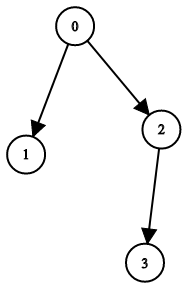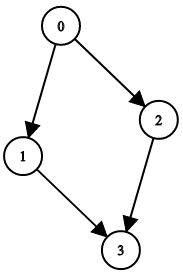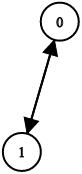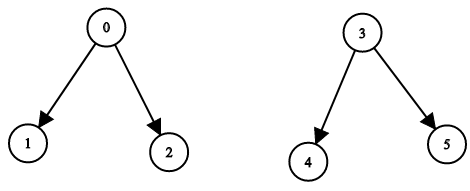# GeetCode Hub

You have `n` binary tree nodes numbered from `0` to `n - 1` where node `i` has two children `leftChild[i]` and `rightChild[i]`, return `true` if and only if all the given nodes form exactly one valid binary tree.

If node `i` has no left child then `leftChild[i]` will equal `-1`, similarly for the right child.

Note that the nodes have no values and that we only use the node numbers in this problem.

Example 1:```Input: n = 4, leftChild = [1,-1,3,-1], rightChild = [2,-1,-1,-1]
Output: true
```

Example 2:```Input: n = 4, leftChild = [1,-1,3,-1], rightChild = [2,3,-1,-1]
Output: false
```

Example 3:```Input: n = 2, leftChild = [1,0], rightChild = [-1,-1]
Output: false
```

Example 4:```Input: n = 6, leftChild = [1,-1,-1,4,-1,-1], rightChild = [2,-1,-1,5,-1,-1]
Output: false
```

Constraints:

• `1 <= n <= 104`
• `leftChild.length == rightChild.length == n`
• `-1 <= leftChild[i], rightChild[i] <= n - 1`

class Solution { public boolean validateBinaryTreeNodes(int n, int[] leftChild, int[] rightChild) { } }